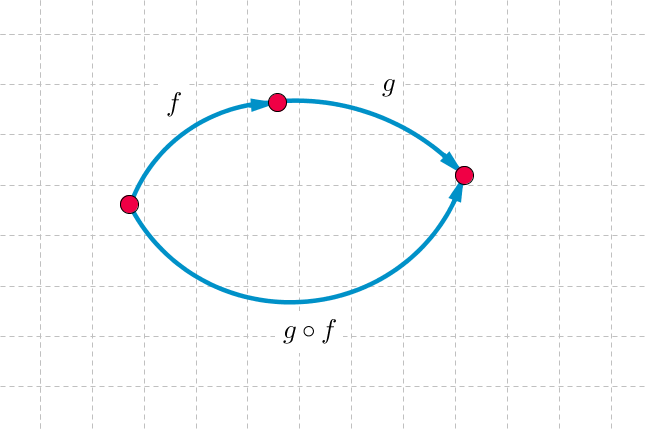## Want to keep learning?

This content is taken from the UNSW Sydney's online course, Maths for Humans: Inverse Relations and Power Laws. Join the course to learn more.
2.9

## UNSW SydneyFirst f, then g.

# Composing functions

When we view graphs as functions, taking a given value of $$\normalsize{x}$$ and outputting a particular value of $$\normalsize{y}$$ according to a rule $$\normalsize{y=f(x)}$$, then an interesting new operation arises: composition.

In this step we look at composing functions rather generally, and give examples from the various kinds of linear, quadratic and inverse functions we have already studied.

## Composing functions

If $$\normalsize{f}$$ and $$\normalsize{g}$$ are functions, then the composition $$\normalsize{g \circ f}$$ is the function defined by:

$\Large{(g \circ f)(x)=g(f(x))}.$

It means that we first apply $$\normalsize{f}$$ to $$\normalsize{x}$$, and then apply $$\normalsize{g}$$ to $$\normalsize{f(x)}$$. This is one of those cases where it might be more natural to read right to left.

## Composition of linear functions

Let’s do a few examples involving simple linear functions. If $$\normalsize{f(x)=x+3}$$ and $$\normalsize{g(x)=5x-1}$$, then

$\Large{(g \circ f)(x)=g(f(x))=g(x+3)=5(x+3)-1=5x+14}.$

Note that

$\Large{(f \circ g)(x)=f(g(x))=f(5x-1)=(5x-1)+3=5x+2}$

and that this is different from $$\normalsize{g \circ f}$$.

We can also note that more generally the composition of two linear functions will be another linear function.

Q1 (E): If $$\normalsize{h(x)=x-1}$$ and $$\normalsize{k(x)=3x+2}$$, find $$\normalsize (h \circ k)(x)$$ and $$\normalsize (k \circ h)(x)$$.

However this is not so simple when we look at compositions of higher degree functions.

If $$\normalsize{f(x)=x^2+1}$$ and $$\normalsize{g(x)=3x^2-x}$$ then

\Large{\begin{align}(f \circ g)(x)=f(g(x)) & =(3x^2-x)^2+1 \\ & =9x^4-6x^3+x^2+1.\end{align}}

In general we can see that the composition of two quadratic functions is going to be a quartic, or degree four, function.

However the composition of a quadratic function with a linear function will be a quadratic function.

Q2 (E): What is the composition $$\normalsize{f \circ g}$$ of the functions $$\normalsize{f(x)=x^2+1}$$ and $$\normalsize{g(x)=3x-4}$$?

Q3 (M): You buy a new kitchen table. Taxes are $$\normalsize{15\%}$$ and delivery is $$\normalsize{\30}$$. If the cost of the table is $$\normalsize{x}$$, then what is your total cost? In this question, there is a bit of an ambiguity, as we are not sure if taxes apply to delivery or not. Suppose we define functions $$\normalsize{t(x)=1.15x}$$ and $$\normalsize{d(x)=x+30}$$. Then what are $$\normalsize{(t \circ d)(x)}$$ and $$\normalsize{(d \circ t)(x)}$$? If taxes don’t apply to delivery costs, then which composite represents your total cost?

Q4 (C): Compositions are not always defined, so one has to be careful. Let $$\normalsize{f(x)=-x^2}$$ and $$\normalsize{g(x)=\sqrt{x}}$$. For which values of $$\normalsize x$$ are the compositions $$\normalsize{(f \circ g)(x)}$$ and $$\normalsize{(g \circ f)(x)}$$ defined?

Q5 (C): The morning operations $$\normalsize t$$ for “brushing teeth” and $$\normalsize e$$ for “eating breakfast” can be performed as $$\normalsize t \circ e$$ or $$\normalsize e \circ t$$. Daniel believes that the correct order of operations is $$\normalsize t \circ e$$ because it keeps teeth clean for longer. Norman reckons that breakfast tastes better when we choose the order $$\normalsize e \circ t$$. What do you think?

A1. The compositions are:

$\Large (h \circ k)(x) = h(3x+2) = ((3x+2)-1) = 3x+1$

and

$$\Large (k \circ h)(x) = k(x-1) = 3(x-1)+2= 3x-1$$.

A2. The composition $$\normalsize{f \circ g}$$ is

$\Large{(f \circ g)(x)=f(3x-4)=(3x-4)^2+1=9x^2-24x+17.}$

A3. The compositions are:

$\Large{(t \circ d)(x)=t(x+30)=1.15(x+30)=1.15x+34.5},$ $\Large{(d \circ t)(x)=d(1.15x)=1.15x+30.}$

If taxes don’t apply to delivery costs, then the total cost is given by $$\normalsize{(d \circ t)(x)}.$$

A4. The composition $$\normalsize{(f \circ g)(x)}$$ can be defined for all positive numbers $$\normalsize{x}$$, since

$\Large{(f \circ g)(x)=f(\sqrt{x})=-(\sqrt{x})^2=-x.}$

However, the composition

$\Large{(g \circ f)(x) = g(-x^2) = \sqrt{-x^2} }$

can be defined only for $$\normalsize{x=0}$$, since the value of the function $$\normalsize{f}$$ is otherwise negative and we cannot take the square root of a negative number.

A5. Let’s take a vote in the discussion.